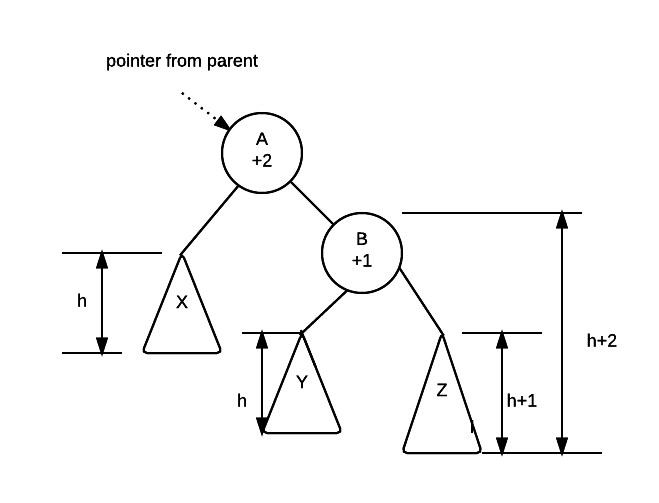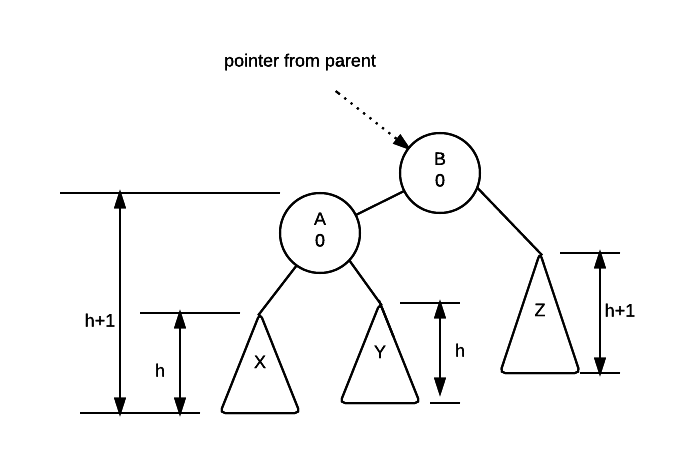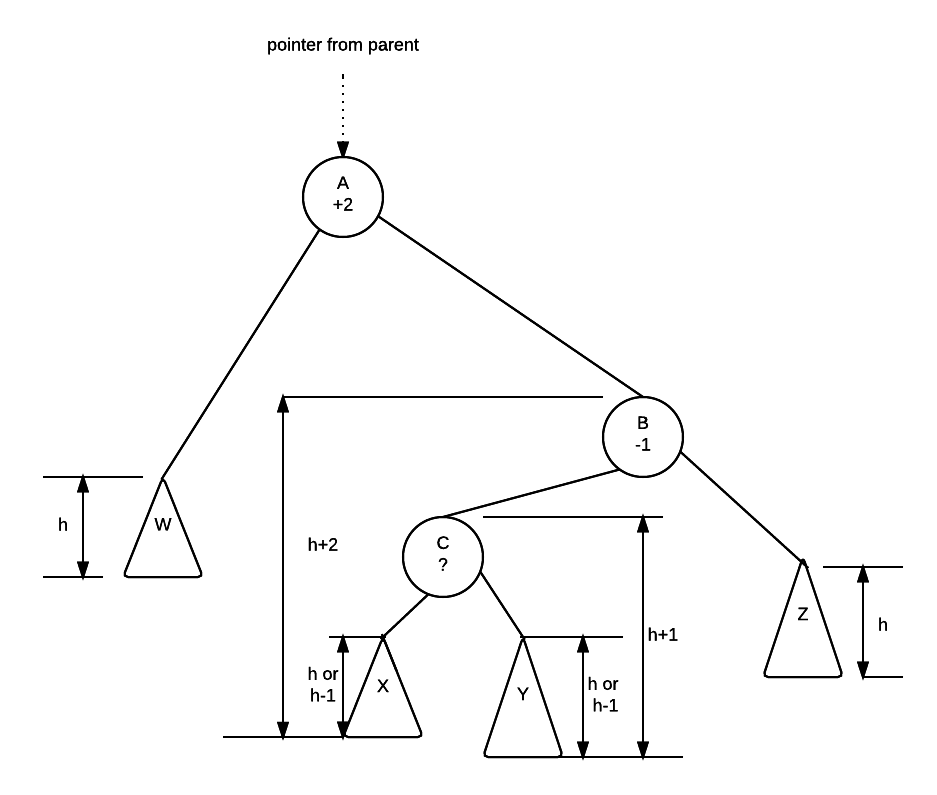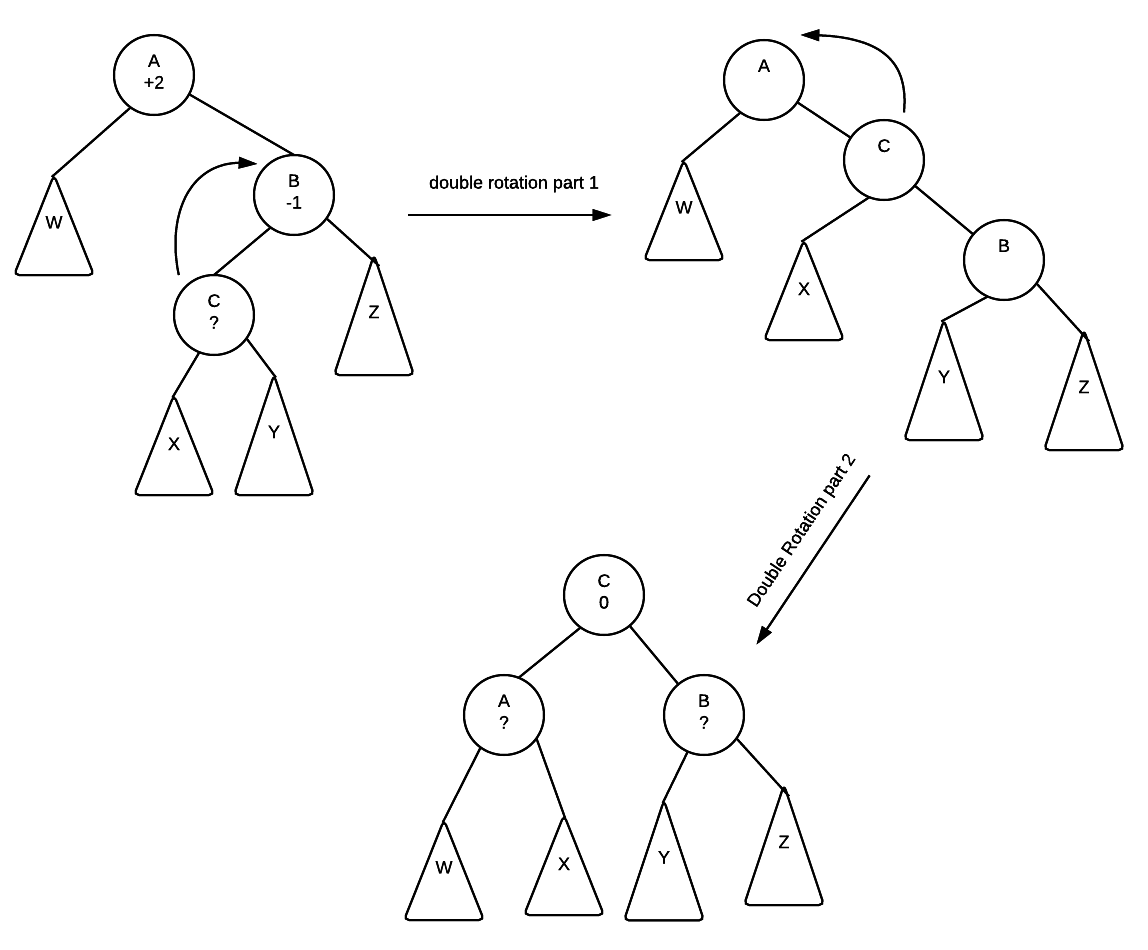# Why it works

## Single Rotation

We always fix nodes starting from the insertion point back to the root. Thus, any node in the insertion path further towards leaf nodes must already be fixed.

Consider the following idea of what an avl tree looks like:In this diagram, we have two nodes A and B and we see their height balance. We know that the subtrees X, Y and Z are valid avl trees because they would have been fixed as we process our tree back to the root.

From here we also know that:

all values  <  A  <  all values  <  B  <  all values
in X                     in Y                in Z


While we don't know how tall X, Y and Z are, we know their relative heights because we know the height balance.

Thus, if X has a height of h, B must be h+2 tall because the height balance at A is +2. This means that the right subtree at B is 2 taller than A.

Continuing on, we know that Z is the taller of the two subtrees of B because the height balance is +1, and thus Z must be h+1 tall while Y must be h tall.

A rotation repositions B as the root of the tree, makes node A the left child of B. Make X the right child of A.

Given that X, Y and Z are unchanged, the height balance at A and B will become 0.The mirror of the above is true for single left rotation

## Double Rotations

A similar explanation of why double rotations work can be reasoned out by looking at the following tree:We know that W, X, Y and Z are all valid avl trees. We also know the following about the values in each of the trees and nodes

all              all               all          all
values  <  A  <  values  <  C  <  values < B <  values
in W              in X             in Y          in Z


Now... we know the height balance of A and B (off balance node and child) we do not know the exact height balance of C. However, we do know that it is a valid avl tree, so C's height balance must be either -1, 0 or +1.

So, if C's height balance is 0, then both x and y will have height of h.

if C's height balance is +1 then y will be h and x would be h-1

if C's height balance is -1 then x would be h and y would h-1

Perform a double rotation:After the rotation is completed, notice that the position of the subtrees W,X, Y and Z along with the nodes A, B and C are placed in a way where their ordering is properly maintained.

Furthermore, The height balance of C becomes 0 regardless of what it was initially. The final height balance of A and B depends on what the original height balance of C was:

original height balance of C height of X height of Y final height balance of A final height balance of B
-1 h h-1 0 +1
0 h h 0 0
+1 h-1 h -1 0